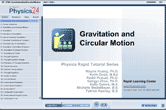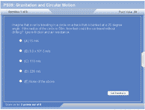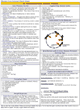How to Learn in 24 Hours?

 Need Help? M-F: 9am-5pm(PST): Toll-Free: (877) RAPID-10 or 1-877-727-4310 24/7 Online Technical Support: The Rapid Support Center vip@rapidlearningcenter.com Secure Online Order:Need Proof? Testimonials by Our Users

 Rapid Learning Courses: MCAT in 24 Hours (2021-22) USMLE in 24 Hours (Boards) Chemistry in 24 Hours Biology in 24 Hours Physics in 24 Hours Mathematics in 24 Hours Psychology in 24 Hours SAT in 24 Hours ACT in 24 Hours AP in 24 Hours CLEP in 24 Hours DAT in 24 Hours (Dental) OAT in 24 Hours (Optometry) PCAT in 24 Hours (Pharmacy) Nursing Entrance Exams Certification in 24 Hours eBook - Survival Kits Audiobooks (MP3)

 Tell-A-Friend: Have friends taking science and math courses too? Tell them about our rapid learning system.Home »  Physics »  College Physics

Circular Motion and Gravitation

 Topic Review on "Title": Univeral law of gravitation: The mathematical formula that relates the pull of gravity between two massive objects that are some distance apart.  Fg=Gm1m2/d2 Gravitation constant: A proportionality constant that relates the strength of gravitational attraction in Newton’s law of universal gravitation. G=6.67x10-11Nm2/kg2  This value always remains constant.  Gravitational field:  The map of influence that a massive body extends into space around itself.  Rotational speed:  Number of rotations or revolutions per unit of time, often measured in rpm, revolutions per minute.  Linear speed is the straight path distance moved per unit of time, also referred to as tangential speed. Centripetal acceleration: The acceleration that describes the change in direction for an object in a circular path. Centripetal force: A center seeking force for an object moving in a circular path. Centrifugal force: An apparent, but nonexistent, outward pointing force for an object moving in a circular path. A rotating object may seem to be pushed outward, but actually must be pulled inward in order to maintain any circular path.

Rapid Study Kit for "Title":
 Flash Movie Flash Game Flash Card Core Concept Tutorial Problem Solving Drill Review Cheat Sheet"Title" Tutorial Summary : Gravity is a force that you notice almost everyday.  This tutorial explains how to calculate or quantify that force.  The gravitational inverse square law depends upon the masses involved, and the distant between them.  A universal gravitational constant is also needed. In previous tutorials, motion in a line was extensively described.  However, motion in a circle is also very common.  The rate at which an object moves in a circular path is described by rotational speed.  The rate at which its direction changes in that circular path is called centripetal acceleration.  The force that provides this acceleration is called centripetal force.  For motion in a circle, the dirction of motion is main quantity that will be described.

 Tutorial Features: Specific Tutorial Features: • Problem-solving techniques are used to work out and illustrate the example problems, step by step. • Vector diagrams showing force components for a car traveling around a banked curve. Series Features: • Concept map showing inter-connections of new concepts in this tutorial and those previously introduced. • Definition slides introduce terms as they are needed. • Visual representation of concepts • Animated examples—worked out step by step • A concise summary is given at the conclusion of the tutorial.

 "Title" Topic List: Gravity Formula Gravitational constant Gravitational fields Gravitational pull at various points on Earth Circular Motion Linear speed versus rotational speed Centripetal acceleration Centripetal force Centrifugal force Banked roads Simulated gravity

See all 24 lessons in College Physics, including concept tutorials, problem drills and cheat sheets:
Teach Yourself College Physics Visually in 24 Hours